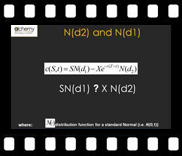# Understanding N(d1) & N(d2) – Course Outline

 The “Understanding N(d1) and N(d2)” course is a video based online course that provides a theoretical and practical overview of the risk-adjusted probabilities of the Black-Scholes option pricing formula.The theoretical overview considers the various elements underlying the Black-Scholes European call option formula, whereas the practical application involves the creation of a Monte Carlo simulation based model in Excel to further clarify these concepts.

# Course Prerequisites

Comfortable with basic mathematics, statistics, probability and EXCEL and some familiarity with derivative products and pricing.

# Course Audience

The course is targeted at basic and intermediate users and is aimed at individuals who deal with pricing, valuation and risk issues related to structured fixed income and foreign exchange transactions.

# Course Guide

Here is the structure of the course.

 Title Duration Session 1 – Black Scholes, N(d1) and N(d2), Monte Carlo Simulator – Theory and Model Review 37:16 mins Session 2 – Monte Carlo Simulator – Basic Model Walkthrough 10:39 mins Session 3 – Understanding N(d1) and N(d2) and Option Exercise using Monte Carlo 15:45 mins

### Session One – Black Scholes, N(d1) and N(d2), Monte Carlo Simulator – Theory and Model Review

The risk training course for understanding risk adjusted probabilities of the Black Scholes equation, N(d1) and N(d2), begins with a power point presentation of the theory setting the foundation for appreciating the difference between N(d1) and N(d2). We start with the Black Scholes European call option formula and move on to primary elements of the underlying process behind the generator function for stock prices, drift and diffusion. We address the concept of risk neutrality and conduct various thought experiments around drift and diffusion to understand their impacts on the plot of stock prices over time. We present three interpretations of the intuition behind the Black Scholes European call option formula and then given a preliminary overview of how to create a Monte Carlo simulation model of the Black Scholes solution in Excel.View a sample of session one

### Session Two: Monte Carlo Simulator – Basic Model Walkthrough

In this part we move from our the theory presented in the power point presentation earlier to the practical application of the understanding the difference between N(d1) and N(d2). We given an overview of the Monte Carlo Simulation model built discussing the input cells including the price path generated, the results from the simulation model and the Black Scholes formula, and the results warehouse where results of 30 simulated runs of the model are stored.

A brief review of how the simulator can be used to generate and store results and how results could be updated by running multiple iterations.View a sample of session two

### Session Three: Understanding N(d1) and N(d2) and Option Exercise using Monte Carlo

After having presented the theory behind the process and a general overview of the various elements of the Monte Carlo simulation model together with the procedure for running the model and updating results in our previous finance videos, we now give a thorough walkthrough of the EXCEL sheet of our Monte Carlo simulation model. We begin with how the results of the payment of exercise price and contingent receipt of stock components of the closed form Black Scholes European call option formula are calculated in the model. Each calculation cell is described in detail, stating precedent and dependent cells, to help with an understanding of:

• How the model is built,
• Difference between N(d1) & N(d2),
• Calculation of the call option value by using Black Scholes formula within the Monte Carlo simulation approachView a sample of session three

## Random Testimonial

• ~ Student Testimonials

"Concise, to the point. Very informative and very practical.”

“I loved the course!! Very interesting. Good lectures.”

“Excellent, very informative and inspiring.”

“In total awe of the way he taught…”

“Well versed with the subject and able to transmit the subject properly.”

“Excellent. If the time could be extended this would be an even better learning experience.”

Excellent teaching skills. The instructor made every effort to explain most complicated finance concepts in simplest possible ways."